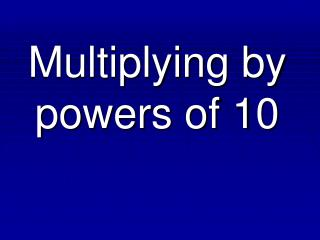DownloadDownload PresentationMultiplying by powers of 10

# Multiplying by powers of 10

Télécharger la présentation## Multiplying by powers of 10

- - - - - - - - - - - - - - - - - - - - - - - - - - - E N D - - - - - - - - - - - - - - - - - - - - - - - - - - -
##### Presentation Transcript

1. Multiplying by powers of 10

2. What are powers of 10? 100,000 10,000 1,000 100 10 1 .1 .01 .001 .0001 .00001 We will only look at these numbers today! GREATER THAN ONE LESS THAN ONE

3. Look for a pattern. 1.24 x 1 = 1.24 1.24 x 10 = 12.4 1.24 x 100 = 124 1.24 x 1,000 = 1,240 1.24 x 10,000 = 12,400 RIGHT The decimal point moves to the ______________ the same number of places as the number of zeros in the power of 10 greater than one.

4. Examples 35 0.35 x 100 = 8.5 x 1000 = 2.72 x 1000 = 5.98 x 10,000 = 8,500 0 0 2,720 0 59,800 0 0

5. WRITE THIS DOWN! If the power of 10 is………….. • MORE THAN ONE You are almost done!  Decimal moves RIGHT

6. Let’s test your knowledge! HOMEWORK Page 143 #9-20, 52-53 25 0.25 x 100 = 6.55 x 100 = 0.9 x 10 = 3.48 x 10 = 9. 655 10. You are the BEST! 9 11. 12. 34.8

7. Look for a pattern. 12.4 x .1 = 1.24 12.4 x .01 = .124 12.4 x .001 = .0124 12.4 x .0001 = .00124 12.4 x .00001 = .000124 LEFT The decimal point moves to the ______________ the same number of digits as there are after the decimal in the power of 10 less than one.

8. Examples . 0 5.4 x 0.01 = 93.6 x 0.1 = 7.84 x 0.001 = 0.3 x 0.0001 = 0.054 . 9.36 . 0.00784 0 0 . 0.00003 0 0 0